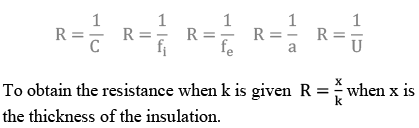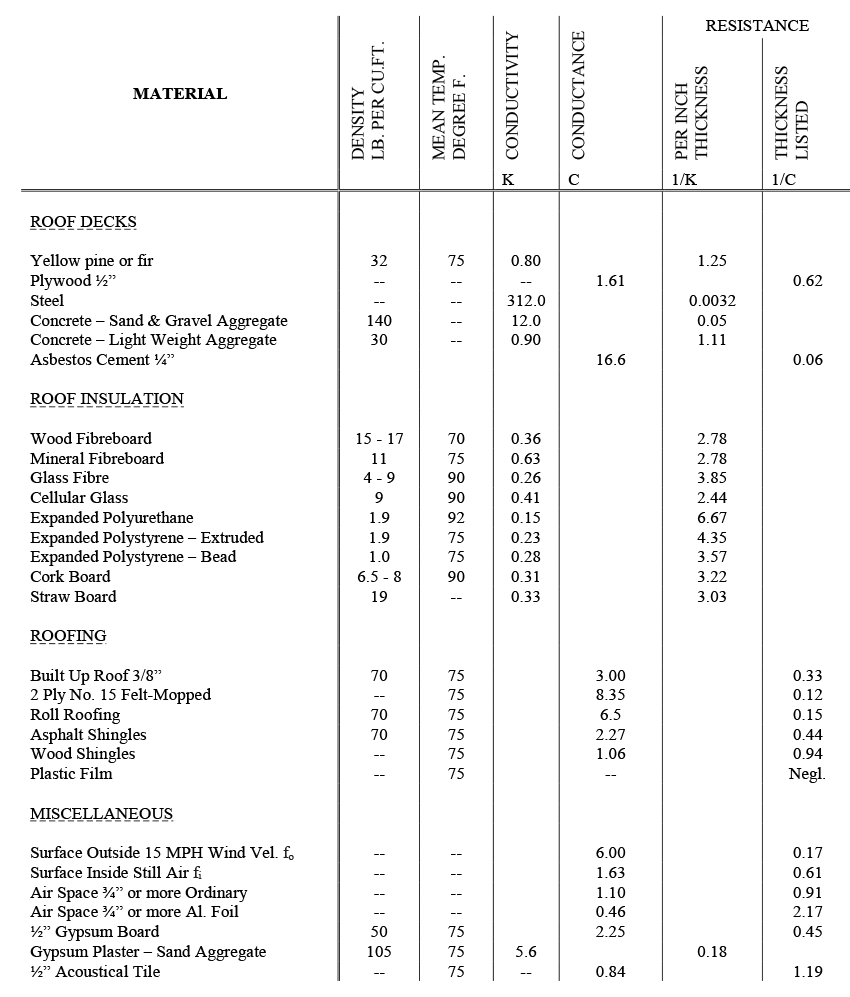Common Technical Terms & Quantities Used In Calculating Insulation Values

May 1969

During any technical discussion on roofing components or assemblies invariably certain technical terms, such as “K” factor “U” value, find their way into the discussion. Frequently these terms are not fully understood and are often misused. In order to clarify their meaning, we list the most commonly used terms  below.

Btu = the unit of heat in the English system is the British Thermal Unit, (abbreviated Btu). which is the amount of heat required to raise one pound of water one degree Fahrenheit, from 63° to 64°. This unit represents a definite and fixed quantity of heat, just as the foot is a unit of linear measure, the pound a unit of weight.

k = thermal conductivity and is the amount of heat expressed in Btu transmitted in one hour through one square foot of a homogeneous material one inch thick for a difference in temperature of ne degree Fahrenheit between the two surfaces of the material. Note, the value of k will vary with the mean temperature. The value of k will vary with the density of the material when the mean temperature is constant. Therefore the density and mean temperature are usually given when expressing the k factor for any insulating material.

C = thermal conductance and is the amount of heat expressed in Btu transmitted in one hour through one square foot and is applied to a specific material as used, which may be either  homogeneous or heterogeneous, for the thickness or type under consideration, for a difference in temperature of one degree F. between the two surfaces of the material.

U = overall coefficient of heat transmission and is the amount of heat expressed in Btu transmitted in one hour per square foot of wall, floor, roof or ceiling assembly for a difference in temperature of one degree Fahrenheit between the air on the inside and outside of the wall, floor, roof or ceiling.

f = film or surface conductance and is the amount of heat expressed in Btu transmitted from a surface to the air surrounding it or vice versa, in one hour per square foot of the surface for a difference of one degree Fahrenheit. To differentiate between inside and outside surfaces, fi is used to designate the inside film and fo the outside film. fi for ordinary building materials and for still air is about 1.63 and fo for ordinary building materials and a wind of 15 miles per hour is about 6.00.

a = thermal conductance of an air space, and is the amount of heat expressed in Btu transmitted in one hour through an area of one square foot of an air space for a temperature difference of one degree Fahrenheit. “a” for an air space of 3/4″ or larger and bounded by ordinary surfaces (not reflective), has an average of approximately 1.1.

R = resistance and is numerically equal to the reciprocal of the conductanceTHE OVERALL RESISTANCE OF A WALL, FLOOR OR ROOF ASSEMBLY IS THE SUM OF THE RESISTANCES OF EACH PART.NOTE:    The above date reflects generally accepted values as published in various industry guide manuals.  For close calculation consult specific suppliers.